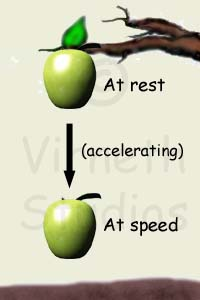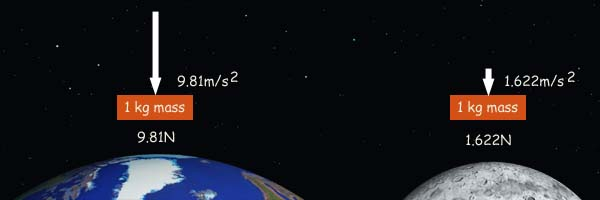# Gravity

Gravity
Weight

## What is Gravity?

Gravity is a force that attracts objects to one another. All matter (what everything is made of) has this attractive force. Gravity is proportional to mass (the amount of matter), which means that as the mass gets bigger so does its gravity by the same rate.

## How do we know Gravity is a force?

The famous 17th century mathematician and scientist Isaac Newton is said to have discovered gravity when he saw an apple drop from a tree.

This may or may not be true; Newton spent a lot of his life studying celestial mechanics, the orbits of planets, and knew there was something keeping the planets in orbit around the sun.

What is important to note is that the apple was at rest in the tree and hit the ground at speed. From his other work Newton realised that the apple must have undergone an acceleration and the only thing that causes a body to accelerate is a force (Force = mass x acceleration).

He named this force Gravity.## How fast does Gravity accelerate things?

At sea level on Earth gravity accelerates mass toward the center of Earth at 9.81m/s2

but that's not a constant -

The strength of gravity is based on two things:

• The amount of mass

Every tiny piece of matter has a gravitational pull on every other piece of matter. When there is a lot of matter together the combined mass has a bigger gravitational pull. In the case of planets, the mass assumes a nearly spherical shape and the gravity appears to be focussed at the centre of it.

• The distance from the centre of the mass

Gravity is subject to the inverse square law.

This means that an object's gravity is weaker further away from the object by a factor that is equal to the square of the distance. For example:

2 times farther away, the gravity is 4 times less;

5 times farther away, the gravity is 25 times less;

10 times farther away, the gravity is 100 times less.

but it never disappears to zero. Your body will be having an effect on every star you can see in the night sky, and even on all those you can't see. In fact you affect the whole of eternity, down to the very last bit!

How Apollo made it to the Moon

## If you want a little more of a challenge -

The gravitational force can be found by using the expression: F = G x (m1 x m2)/r2 = (G x (m 1/r2)) x m2

Where

G is the Universal constant of gravitation 6.67 x 10−11 kg−1 m3 s−2

m1 and m2 are two masses

Newton's second law of motion tells us that F = m x a

so G x (m1 x m2)/r2 = m2 x a

eliminating m2 from both sides by division leaves us with

## a = G x (m1/r2)

where a = acceleration due to gravity at radius r from the centre of mass m1

This only really works for planets because they are spherical and their mass appears to be concentrated at the centre.

It is also an approximation - in reality gravity changes all over due to factors like local concentrations of mass, such as the Himalayas.

Try putting in 5.97 x 1024kg for the mass of the Earth and 6.378 x 106m for the radius at sea level.

You should get something close to 9.81 m/s2, which is the accepted value.

# Mass and Weight

Mass is not Weight

## Mass

Mass is the amount of matter in a body. The mass remains the same wherever the body is. It would be the same on the Earth as on the Moon

## Weight

Weight is the force that mass experiences due to acceleration.

Weight is measured in Newtons just like any other force.

Gravity is a force that causes objects to accelerate.

Generally, we use Newton's Second Law of Motion to find the weight: F = m x a

Where F is the weight, m is the mass of the body and a is the acceleration due to gravity (9.81 m/s2 at sea level on Earth).

The mass of the Moon is about 1/6 that of the Earth so the gravity isn't as strong. This means that a mass of 1kg would weight much less on the Moon than on Earth.

The acceleration due to gravity on the moon is 1.622 m/s2 (or 0.1653g as a ratio of Earth's gravity).## Weightlessness

Gravity is everywhere in the Universe so there is no escaping its force; there is no such thing as zero gravity. The only way a body can be weightless is when the forces of gravity are balanced. For example a position between Earth and the Moon.

Drag the 1kg mass back and to between Earth and the Moon to see how the weight changes

'But astronauts are weightless; they travel around the Earth and don't stay in one special position' you say!

Astronauts and their spacecraft are actually falling due to gravity. It's just that they never land so there is no upwards force from the ground to give the feeling of weight.